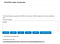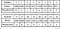# Hash functions explained in a simple way

## Understanding how hash functions work

Simply speaking, a hash function is a mathematical function that takes any input size and convert it to a fixed output size. Consider this simple hash function H(X) = Last digit of (X)

• H(1234) = 4.
• H(12567) = 7.
• H(127) = 7.
• H(1111111111) = 1.
• H(24)=4.
• H(24)=4.

So no matter what is the input and its size, we’re returning a one digit output. Another important property is that the same input will always give you the same output. H(24) will always be 4.

In summary:

• A hash function generates a fixed size output (called digest or hash)
• For any input size or length, we get always the same fixed output size
• Totally deterministic: for the same input we get always the same output
• It’s not a complicated operation to get the hash of any input (very easy)
• Several inputs can have the same hash (like here 127 and 12567 both have 7 as hash). That’s called a hash collision. It’s natural since the output has a fixed size, and the input can be of ANY size, there are infinite inputs for a fixed number of outputs. For this hashing example, there can be only 10 possible outputs (1 digit — from 0 to 9). So it will be super easy to find a hash collision, giving there are infinite numbers as inputs.

The first example I gave you, is not really a cryptographic hash function, why? because you can easily reverse it. AKA: finding an input for a given output.

Basically if i tell you what input gives a hash of 6? Any number ending with 6 is a valid answer. Simple: 123456 is a candidate, 556 is another candidate. 96 is a third candidate. The ability to reverse a hash function is a bad Cryptographic property.

So cryptographic hash functions have, in addition, the following properties:

• It should be very hard, starting from a certain output, to get back one of the valid inputs.
• It should be very hard and rare to find a collision (2 inputs generating the same hash)

One famous cryptographic hash functions is the SHA256. For any input size, it generates 256 bits of digest. It’s so easy for any input to get the output, you can test it yourself here: https://passwordsgenerator.net/sha256-hash-generator/Test1234 has a hash 07480FB9E85B9396AF06F006CF1C95024AF2531C65FB505CFBD0ADD1E2F31573

Go to this website and try the text Test1234 with capital “T”, you should get the following hash: 07480FB9E85B9396AF06F006CF1C95024AF2531C65FB505CFBD0ADD1E2F31573

Anyone can easily validate that the text “Test1234” has actually that hash.

Note that the hash is written using 64 characters just as a compact way to represents the 256 bits. This is called HEX representation, standing for hexadecimal base. The way it works is the following:Hex to binary

You have 16 characters in hex base ( the 0 -> 9 are 10 characters +6 letters: A,B,C,D,E,F = 16 characters in total), each can encode one of the combination of 4 bits.

So when you get 64 hex for sha256, you’re getting directly 64 x 4 = 256 bits.

As you can see, a 0 in hex is actually 0000 in binary. 7 is 0111. So the hash of Test1234 is the following in binary:

Replace 0 by 0000. Replace 7 by: 0111. Replace 4 by 0100. Replace 8 by: 1000. Replace 0 by 0000. Replace F by 1111. Replace B by 1011…

So instead of : 0 7 4 8 0 F B … in Hex

you get: 0000 0111 0100 1000 0000 1111 1011 … in Binary

In summary, each hex digit is just a direct mapping of 4 bits in binary.

A secure hash function should act like a random number generator. So if you change a tiny bit the input, half of the bits will change randomly at the output.

Actually even just changing one character from the input. Let’s say we try test1234 with small t instead of capital T.

You get the following hash of test1234:

937E8D5FBB48BD4949536CD65B8D35C426B80D2F830C5C308E2CDEC422AE2244

Nothing to do with the first hash of Test1234

07480FB9E85B9396AF06F006CF1C95024AF2531C65FB505CFBD0ADD1E2F31573

So if a hash is very secure that it mixes well the bits before you get an output, it will cost you 2^bits to break the hash.

So for 256 bits hash, you would need to try 2²⁵⁶ times that’s equal to 1.1x10⁷⁷ tries before you find an input (Almost the number of atoms in the universe!)

If you don’t believe me, here is a challenge for you: try to find the text that generates the following hash, go to this website and try, type any random text as input, and see how close you can get to the following hash:

76B7EA4ADA84E973F394C9F5E6CDA618FC6CC246B2130B0BD2FE6092493D84A4

I would say, good luck! If you just get the starting 76B7E you would be very lucky! Let me know in the comments how many hex you manage to break?

Interested in artificial intelligence, machine learning, neural networks, data science, blockchain, technology, astronomy. Co-founder of Datathings, Luxembourg

## More from Assaad MOAWAD

Interested in artificial intelligence, machine learning, neural networks, data science, blockchain, technology, astronomy. Co-founder of Datathings, Luxembourg